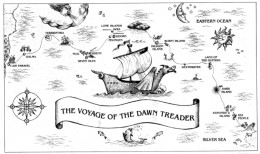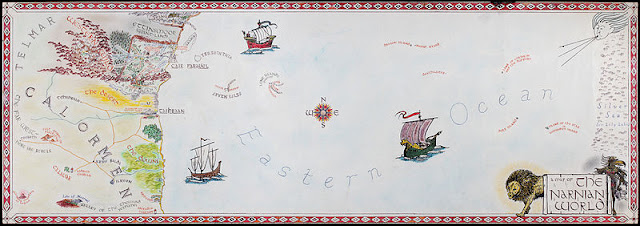## Tuesday, March 19, 2013

### The Width of NarniaAs is revealed in Voyage of the Dawn Treader, the world of Narnia is a flat earth.  Characters can literally fall of the edge of the world.

I started wondering, at one point, how thick is that edge?

Characters from our world report no differences in the gravity (or whatever) on Narnia; they don't feel any greater or lesser weight walking around.  Arguably, if Narnia had a lower gravity, then the Pevensies might have had, at least, an easier time crossing through the snow.  And contrariwise, if Narnia had a much higher gravity, then the adventure would have mostly been about aching knee joints.

Further, when the Pevensies stay in Narnia as kings and queens, they eat the food there, and this does not make them sick.  The food they eat they report as tasting equivalent to earth food.  When they grow up, they marry dryads and naiads and other mythological things and have children.  Weird as this is, it all proves pretty much conclusively that Narnia is made of the same kind of "stuff" as Earth; this is important.

So we know three things.  We know Narnia has the same overall downward-pulling force as Earth's gravity, we know that Narnia is made of the same kind of stuff as Earth, and we know that Narnia is a flat earth with a literal edge that you could fall off.

This is enough to calculate, to a very good approximation, how thick Narnia is.

The first approximation that we're going to make, is that dirt in Narnia and dirt in Earth have the same density.  That is, if I had a dump truck full of Narnian dirt and an identical dumptruck full of Earth dirt, the two dump trucks will have the same mass.  As I said, this is reasonable, because Narnia soil is similar enough to support the same flora and fauna as Earth soil.  If it's made of the same stuff, then it can't be too far off from Earth's density.

The second approximation that we're going to make... is that Narnia doesn't have an edge.

This is initially absurd, but just hold on and I'll explain why we make it.

Physicists like to pretend that things are things they are not, because the things they are not are easier to talk about.  Typically, the differences aren't really essential to understand the system in question.  A common physics joke is assuming a spherical cow; we want to take in to account the fact that cows have shape to include something like air resistance... but cows are really a really complicated shape, so we go with a sphere.

It's kind of the same here.  The important property is the fact that Narnia is flat, not that it's flat and finite.  So for instance, if you are standing in in Lantern Waste, the edge of the world is very, very far away.  Months away by boat.  What happens at the edge is basically that the gravitational field won't be quite perfectly up/down (it will actually try to pull you back towards the center) and that it will be weaker than elsewhere; but if you're in Lantern Waste, what happens at the edge is so far away that it really doesn't matter.It's a long way from Lantern Waste to the edge; so long that edge effects "won't matter".

We could calculate things explicitly taking in to account that Narnia is finite in extent... but the answer we got wouldn't actually be much different.  At least not in Lantern Waste.

It's also worth noting, I am assuming that the downward-pulling-force of Narnia is gravity, the actual Earth gravity we all know and love, and I'm ignoring general relativistic weirdness and just assuming all gravity follows the simple formulation of Newton.  It is very likely that what keeps people on the ground in Narnia is not actually gravity, but something else, something different that we've never heard of and will never think of in our wildest imaginations -- for instance, if Narnia worked with normal gravity, then it should crumple up rather quickly in to a sphere.  Sadly, since I don't know what the downward-pulling force of Narnia is nor how to describe it, I can only go with what I've got.

Newton's equation for gravity is
$$\mathbf{g} = -G \int \frac{\rho}{r^2}\hat{\mathbf{r}} dV,$$
where $\mathbf{g}$ is the gravitational field of earth, $\rho$ is the mass-density of the object in question, and $r$ is the distance from that object to where we are.  Wherever we are, we could just integrate this over the entire world of Narnia to find the gravitational field there.  It'd be kind of difficult.

In the real world with real gravity, for special highly symmetric systems (like, say, infinite flat planes), we can perform a really cool trick that exploits the symmetries to solve for an answer, without needing to do tedious integrations.  This trick is called Gauss' Law.

So assume Narnia is an infinite flat plane of some width $w$ with mass-density $\rho$, where $\rho$ is the mass-density of earth.  The picture I'm thinking of looks something like this:

which I drew just now (author is not professional artist).  Note that I drew an edge -- I can't draw an infinite place.  Use your imagination to pretend it carries on forever in each lateral direction.

Now, because the plane is infinite in extent, the strength of gravity cannot depend on where you are in that infinite plane --- all locations are exactly the same!  If anything, it can only depend on how high you are (it doesn't depend on that, either, but that's a bit less obvious).  Further from symmetry, the direction of gravity can only be up or down.

To find that dependence, we will draw a cylinder-thing of height $h$ above the center of Narnia and height $h$ below the center of Narnia, and total cross-sectional area $A$ (the exact shape of $A$ doesn't matter so long as it's constant).  Something like this drawing, where I've removed all the mountains and vales of Narnia - the cylinder is yellow and the gray part is the part of the cylinder actually enclosing dirt.

Now, from Gauss' law, the gravity flux out of the cylinder is proportional to the amount of mass contained in the cylinder.  That is
$$\iint \mathbf{g} \boldsymbol{\cdot} d\mathbf{A} = -4\pi G \iiint \rho dV.$$
This might look complicated, but it's kind of easy with the symmetry.  The left-hand-side is just $-2gA$, while the right-hand-side is just $-4\pi G Aw\rho.$

Therefore,
$$2\pi G \rho w = g.$$
If we let $M$ be the mass of the earth and $R$ the radius of the earth, then
$\rho = \frac{3M}{4\pi R^3}$ and $g = G\frac{M}{R^2}$, and doing some algebra, we can simplify this to
$$w = \frac{2}{3} R.$$
That is, Narnia is 2/3rds as thick as the radius of the Earth, under the assumptions given.  In miles, that is $2642$ miles.

Which is a pretty long way to fall in a boat.